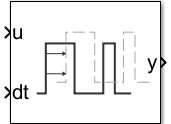# Propagation Delay

Model loop delay, latency, pulse delay

Since R2022b

•Libraries:

## Description

The Propagation Delay block delays the current value for a signal into the future by an amount specified by a delay signal. The Propagation Delay block is well suited for implementing time delay in a discrete system.

On each simulation step k, the block samples the signal input Uk and the delay input Dk. The block output value becomes the signal value after the delay time Dk has elapsed from the current simulation time Tk.

y(t) = Uk when t = Tk + Dk

At the start of the simulation, the block output value is the Initial output block parameter value Y0. The first sample of the delay signal D0 determines when the block output changes from the Initial output value to the delayed input signal values.

y(t) = Y0 when t < T0 + D0

When the simulation reaches the time T0 + D0, the block output is determined by the signal and delay samples.

y(t) = Uk when Tk + DktTk+1 + Dk+1,

where:

• Uk is the sample of the input signal taken at t = Tk.

• Dk is the sample of the delay signal taken at t = Tk.

• Tk+1 is the simulation time at which the next signal and delay samples were taken.

• Dk+1 is the delay sample taken at time Tk+1.

## Ports

### Input

expand all

The Propagation Delay block supports real, scalar input signals with continuous or discrete sample time. The input signal data type can be any built-in type besides `int64` and `uint64`.

Data Types: `single` | `double` | `int8` | `int16` | `int32` | `uint8` | `uint16` | `uint32` | `Boolean`

The delay signal specifies the amount of delay to apply at each time step in the simulation. You can use the Propagation Delay block to implement a constant or variable delay.

The delay signal values must satisfy these constraints:

• The delay signal must contain scalar, real, finite, positive values larger than `128*eps`, which is the smallest time resolution the software supports.

• The output times for delayed input signal samples must increase monotonically.

For example, suppose the delay signal value is `0.6` at simulation time `0` and the next simulation step occurs at time `0.5`. The delay signal at time `0.5` must be greater than `0.1` so that the delayed output time for the input signal sample U0.5 is greater than the output time for input signal sample U0 (`0.6`).

• When you select Run at fixed time intervals, the delay signal values must be greater than the value you specify for the Sample time parameter.

Data Types: `double`

### Output

expand all

The output signal has the same data type as the input signal. By default, the output signal has fixed-in-minor-step sample time. When you select Run at fixed time intervals, the output signal has the discrete sample time you specify for the Sample time parameter.

Data Types: `single` | `double` | `int8` | `int16` | `int32` | `uint8` | `uint16` | `uint32` | `Boolean`

## Parameters

expand all

Specify the initial output signal value Y0. The first sample of the delay signal D0 determines when the block output changes from the Initial output value to the delayed input signal values.

y(t) = Y0 when t < T0 + D0

#### Programmatic Use

 Block Parameter: `InitialOutput` Type: string | character vector Values: numeric scalar Default: `0`

This parameter specifies how the block executes and the sample time for the output signal.

• `off` — Block executes each time the delay for an input sample elapses. Output signal has fixed-in-minor sample time.

• `on` — Block executes at a fixed rate that you specify using the Sample time parameter. Output signal has the sample time you specify using the Sample time parameter.

When you select Run at fixed time intervals:

• The delay signal values must be greater than the value you specify for the Sample time parameter.

• Delay signal values that are not integer multiples of the specified sample time are rounded down to the nearest integer multiple of the sample time. For example, if the sample time is `0.1` and the delay signal value is `0.68`, the software rounds the delay to `0.6`.

#### Programmatic Use

 Block Parameter: `RunAtFixedTimeIntervals` Type: string | character vector Values: `'on'` | `'off'` Default: `'off'`

Specify the sample time for the block output signal. The delay signal values must be greater than the specified sample time.

When the delay signal value is not an integer multiple of the specified sample time, the software rounds the delay value down to the closest value that is an integer multiple of the sample time. For example, if the sample time is `0.1` and the delay value is `0.68`, the software rounds the delay to `0.6`.

#### Dependencies

To enable this parameter, select Run at fixed time intervals.

#### Programmatic Use

 Block Parameter: `SampleTime` Type: string | character vector Values: numeric scalar Default: `0.1`

## Block Characteristics

 Data Types `Boolean` | `double` | `integer` | `single` Direct Feedthrough `no` Multidimensional Signals `no` Variable-Size Signals `no` Zero-Crossing Detection `no`

## Version History

Introduced in R2022b